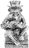### Try to print a view using the code example from nioxus 600.08 with my own filter. Code shown here returns nothing. Any help is appreciated.

let i := null;
let xCurrRec := Id;
let xBeg := 'BEG Date';
let xEnd := 'END Date';
let i := (create 'G-Reports');
i.('S-Date' := xBeg);
for j in select Grants where Date => xBeg and Date =< xEnd do
let k := (create 'Print Lines');
k.(Date := j.Date);
k.('Project Description' = j.'Project Description');
k.(Recipient = j.Recipient);
k.('Lodge Share' = j.'Lodge Share');
k.('Fdn. Share' = j.'Fdn. Share');
k.('G-Reports' := i)
end;
printRecord(record('G-Reports',number(i)), "Grant Detail");
"delete (select 'G-Reports')"At a glance this is what I see...

`Date => xBeg and Date =< xEnd`

should be...

`Date >= xBeg and Date <= xEnd`